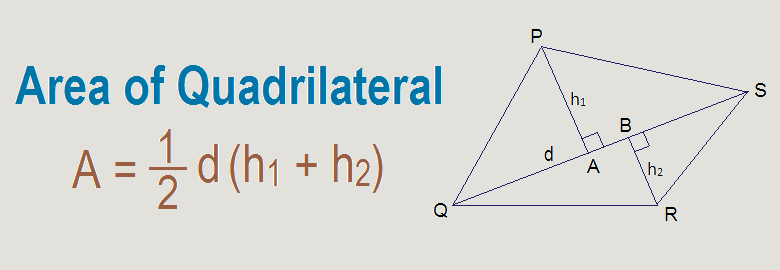Area is the region covered by a closed plane figure. Quadrilateral is a closed plane figure bounded by four sides. Area of quadrilaterals can be measured by using different formula for different types of regular shaped quadrilaterals. They are as given below:

Area of rectangle = l × b, where l = length of rectangle and b = breadth of rectangle
Area of square = l2 or ½ d2, where l = length of square and d = diagonal
Area of parallelogram = b × h, where b = base and h = height
Area of rhombus = ½ × d1 × d2, where d1 and d2 are two diagonals
Area of kite = ½ × d1 × d2, where d1 and d2 are two diagonals
Area of trapezium = ½ × h × (l1 + l2), where h = height and l1 and l2 are two parallel lines

### Area of a irregular quadrilateral

Area of irregular or any shaped quadrilateral can be obtained in terms of its one diagonal and two perpendiculars drawn from the opposite vertices to the diagonal.
In the given figure, PQRS is a quadrilateral in which QS is a diagonal. PA and RB are perpendiculars drawn from the vertex P and R respectively to the diagonal QS. Suppose, QS = d, PA = h1 and RB = h2.
We can write:
Area of quadrilateral PQRS = Area of ΔPQS + Area of ΔRQS
= ½ QS × PA + ½ QS × RB
= ½ d ×h1 + ½ d × h2
= ½ d (h1 + h2)
Area of quadrilateral = ½ d (h1 + h2where, d is a diagonal, h1 and h2 are the perpendiculars drawn from the opposite vertices to the diagonal d.

### Workout Example

Example 1: Find the area of the given quadrilateral.
Solution: From the figure,
Length of diagonal (d) = 14cm
h1 = 5cm
h2 = 4cm
Area of quadrilateral = ½ d (h1 + h2)
= ½ × 14 (5 + 4)
= ½ × 14 × 9
= 63 cm2

You can comment your questions or problems regarding the area of quadrilaterals here.